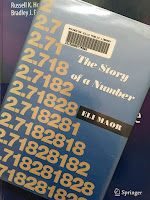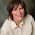## Friday, May 27, 2011

### e, The Story of a Number

On page 33 of the 4th edition of Intermediate Physics for Medicine and Biology, Russ Hobbie and I introduce the constant e.
The number e is approximately equal to 2.71828… and is called the “base of the natural logarithms.” Like π (3.14159…) e has a long history [Maor (1994)].e: The Story of a Number, by Eli Maor.
The citation is to the delightful book e: The Story of a Number, by Eli Maor. In his preface, Maor explains why he wrote the book.
My goal is to tell the story of e on a level accessible to readers with only a modest background in mathematics. I have minimized the use of mathematics in the text itself, delegating several proofs and derivations to the appendixes. Also, I have allowed myself to digress from the main subject on occasion to explore some side issues of historical interest. These include biographical sketches of the many figures who played a role in the history of e, some of whom are rarely mentioned in textbooks. Above all, I want to show the great variety of phenomena—from physics and biology to art and music—that are related to the exponential function ex, making it a subject of interest in fields well beyond mathematics.
Our Chapter 2, about exponential growth, centers on the exponential and logarithm functions, and our Appendix C lists many of the properties of these functions. Maor explores all sorts of interesting facts about e. For instance, 878/323 is a very good rational approximation to this irrational number. You can recall the first ten digits of e by remembering 2.7 (Andrew Jackson)2 [Jackson was elected president in 1828]. In his Chapter 13, Maor presents some beautiful continued fractions for e that I will not attempt to reproduce here using html.

When developing the Fourier series in Chapter 11 of Intermediate Physics for Medicine and Biology, Russ and I note that “the remarkable property of imaginary numbers that make them useful in this context is that e = cosθ + i sinθ.” (Here, i is the square root of minus one.) Maor sets θ = π to obtain an equation studied by the Swiss mathematician Leonhard Euler

e = −1 ,

and claims
it must surely rank among the most beautiful formulas in all of mathematics. Indeed, by rewriting it as eπi + 1 = 0, we obtain a formula that connects the five most important constants of mathematics (and also the three most important mathematical operation—addition, multiplication, and exponentiation). These five constants symbolize the four major branches of classical mathematics: arithmetic, represented by 0 and 1; algebra, by i; geometry, by π; and analysis, by e.
Taking a less aesthetic view, Russ and I downplay the use of complex exponentials in Intermediate Physics for Medicine and Biology.
The Fourier transform is usually written in terms of complex exponentials. We have avoided using complex exponentials. They are not necessary for anything done in this book. The sole advantage of complex exponentials is to simplify the notation. The actual calculations must be done with real numbers.
Another reason I often steer clear of complex exponentials is that I place great importance on being able to visualize physically what a mathematical expression is saying, and I find trigonometric functions far easier to envision than complex exponentials. So, while I concede the abstract beauty of the formula e = −1, I don’t find it so useful when thinking about physics.

While educating his readers about e, Maor also introduces them to many famous mathematicians, including Archimedes, Napier, Newton, Gauss, the Bernoullis, and above all Euler, who is apparently one of Maor’s favorites.
Leonhard Euler (1707–1783) is unquestionably the Mozart of mathematics, a man whose immense output--not yet published in full—is estimated to fill at least seventy volumes. Euler left hardly an area of mathematics untouched, putting his mark on such diverse fields as analysis, number theory, mechanics and hydrodynamics, cartography, topology, and the theory of lunar motion.
Maor discusses the uses of logarithms and exponentials in biology. He talks about the logarithmic spiral and its role in growth, for instance, of a nautilus shell. He also makes an interesting comparison between the ear and the eye.
The remarkable sensitivity of the human ear to frequency changes is matched by its audibile range—from about 20 cycles per second to about 20,000 (the exact limits vary somewhat with age). In terms of pitch, this corresponds to about ten octaves (an orchestra rarely uses more then seven). By comparison, the eye is sensitive to a wavelength range from 4,000 to 7,000 angstroms (10−8 cm)—a range of less than two “octaves.” [Doesn’t Maor mean: less than one “octave”?]
I’m particularly fond of Maor’s recreation of a meeting between Bach and one of the Bernoulli’s
Let us imagine a meeting between Johann Bernoulli (Johann I, that is) and Johann Sebastian Bach. The year is 1740. Each is at the peak of his fame. Bach, at the age of fifty-five, is organist, composer, and Kapellmeister (musical director) at St. Thomas’s Church in Leipzig. Bernoulli, at seventy three, is the most distinguished professor of the University of Basel.
The resulting imagined conversation is fascinating and amusing. Musicians interested in the “equal tempered scale” will enjoy this section.

I will close this blog entry the same way Maor ended his book, by letting e take a final bow. Here is e to one hundred decimal places:

2.7182818284590452353
60287471352662497757
24709369995957496696
76277240766303535475
94571382178525166427

1.2.